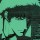## “Presto” math trick in Java

According to Futility Closet, if you start with a 3 digit and place it next to the same number to form a 6 digit number, you can divide the 6 digit number by 7, 11, and then 13 and you will end up with the original 3 digit number and no remainders. For example, by taking the number 412 and making it 412412 and then doing the divisions, you will end up with 412. I wrote a small program in Java to test it.

### The Code

```public class Main
{
public static void main(String[] args)
{
for(int i = 100; i <= 999; i++)
{
//get the number, and "double" it
int number = Integer.parseInt(Integer.toString(i) + Integer.toString(i));
//successively divided by 7, 11, then 13
System.out.print((i) + "t");
System.out.print((number) + "t");
System.out.print((number /= 7) + "t");
System.out.print((number /= 11) + "t");
System.out.print(number /= 13);
//is the result what we expect? (input == output)
if(i == number)
{
System.out.print("tCorrectn");
}
else
{
System.out.print("tIncorrect");
break;
}
}
}
}```

In this snippet, we loop through all 3 digit numbers (100 to 999) and output the results of each operation in a tab separated column. If the result (output) is the same as the input, we print correct and continue with the loop. Otherwise, we print incorrect and break. Give it a try!

1.Daniel Fitzpatrick
2.Daniel Fitzpatrick